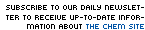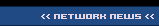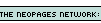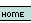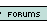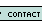1. How many moles in 20 g of water? 1.11 20 18 1.1 42 2. How many carbon molecules are present in 5.0 grams of glucose? (C6H12O6) 1.0 * 10^22 5.1 * 10^22 1.0 * 10^23 6 None 3. When aluminum reacts with sulfuric acid, the result is aluminum sulfate and hydrogen gas. How many moles of sulfuric acid (H2SO4) will react with 24 moles of aluminum? 12 14 16 18 20 4. A chemistry student was performing an experiment involving the following reaction: C3H8 (g) + O2 (g) --> CO2 (g) + H2O (g), balancing it with the coefficients 1, 5, 2, and 4 respectively. The oxygen was in excess. He used 20.18 grams of C3H8. When he measured the amount of CO2 produced, he was astounded to find that 46.22 grams were produced, 5.88 grams more than the theoretical yield of 40.34. What went wrong? Hint: Look at the formula C3H8's coefficient should be 3, not 1 CO2's coefficient should be 3, not 2 O2's coefficient should be 3, not 5 Water's coefficient should be 3, not 4 None of these 5. If 11 grams in a reaction was obtained, and you had a theoretical yield of 14 grams, what is your % yield? 0.79% 79% 11% 14% 12% 6. Substance A combines with substance B to form Substance AB. B is a diatomic molecule. You are given 3.15 moles of B2. If A is in excess, how many moles of AB are produced? 1.20 2.30 3.25 5.60 6.30 7. The empirical formula of a compound is C10H20 with a molar mass of 284 grams, find the molecular weight. C20H40 C10H20 C5H10 C4H8 CH4 8. How many kilometers are in 10.00 miles? (1 inch equals exactly 2.54 centimeters) 2.04 km 4.09 km 8.09 km 16.09 km None of these 9. Hydrochloric acid reacts with calcium carbonate yielding calcium chloride, water, and carbon dioxide. How many moles of calcium carbonate would be necessary to react with 8 moles of HCl? 1 2 3 4 5 10. Substance X has a mass of 36.08 grams. Calculations show that .199 moles of substance X exist. What element is most likely X? Molybdenum Technetium Ruthenium Rhodium Palladium 11. How many grams is 0.25 moles of table salt (NaCl?) 58.44 grams 14.61 grams 14.6 grams 58 grams 15 grams 12. There are two beakers sitting on a table. One contains 56.20 grams of water. The other contains hydrochloric acid (HCl). The number of atoms of hydrogen in the HCl equals the number of total atoms in the beaker full of water. How many grams of HCl are in the second beaker? 341.4 grams 142.4 g 938.1 g 53.29 g None of these 13. When 2 moles of propane (C3H8, MW = 44 grams) is combusted with oxygen gas, how many grams of CO2 (MW: 44 grams) are produced? 88 44 3 132 264 14. 6.02x1023 is.... a random number Avogadro's number stoichiometry percent yield None of these 15. Hydrogen gas and oxygen gas can form water. What mass of water will form from excess oxygen and 24.0 g H2? (Assume a complete reaction) 107 216 24.0 48.0 96.0 Name: E-mail: Last 15 Challengersnews | about us | contact us tutorials index | organic chemistry | practice tests | online quizzes | reference tools site copyright (c) 2002 to neopages.com